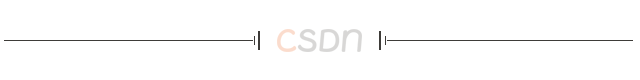【CSDN 编者按】元宵佳节，一起赏花灯。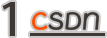Python环境和模块

• numpy

• pillow

• wxgl

``````pip install numpy
pip install pillow
pip install wxgl
``````

NumPy和 pillow 是 Python 旗下最常用的科学计算库和图像处理库，属于常用模块。WxGL 是一个基于 PyOpenGL 的三维数据可视化库，以 wx 为显示后端，提供 Matplotlib 风格的交互式应用模式，同时，也可以和 wxPython 无缝结合，在wx的窗体上绘制三维模型。关于 WxGL 的更多信息，请参阅我的另一篇博客《十分钟玩转3D绘图：WxGL完全手册》https://xufive.blog.csdn.net/article/details/108958992。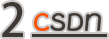``````>>> import numpy as np
>>> from PIL import Image
>>> import wxgl.wxplot as plt
``````

``````>>> fn = r'D:\temp\light0115\res\paper.png'
>>> im = np.array(Image.open(fn))/255
>>> im.shape
(400, 942, 3)
``````

fn定义的是图像存储路径，请据实修改。Image.open(fn)打开文件，返回一个PIL对象，np.array()将PIL对象转成numpy.ndarray数组对象。除以255，将图像数据从0到255的值域范围变成0到1，适应WxGL的接口要求。查看数组的shape，显示图像分辨率为400像素高、942像素宽，每个像素有三种颜色（此处为RGB）。

``````>>> rows, cols, deep = im.shape
>>> cols/(2*np.pi)
149.9239563925654
>>> r = 1
>>> h = 2*np.pi*rows/cols
>>> h
2.6680192387174464
``````

``````>>> theta = np.linspace(0, 2*np.pi, cols)
>>> x = r * np.cos(theta)
>>> y = r * np.sin(theta)
>>> z = np.linspace(0, h, rows)
>>> xs = np.tile(x, (rows,1))
>>> ys = np.tile(y, (rows,1))
>>> zs = z.repeat(cols).reshape((rows,cols))
``````

``````>>> plt.mesh(xs[::10,::10], ys[::10,::10], zs[::10,::10], mode='FLBL')
>>> plt.show()
``````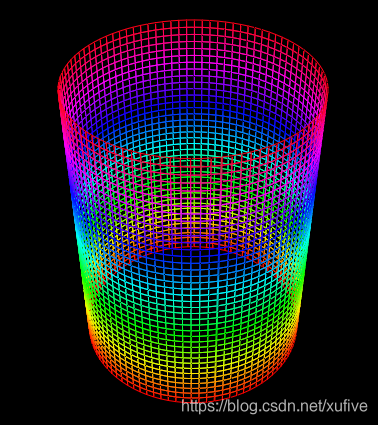``````>>> plt.mesh(xs, ys, zs, im)
>>> plt.show()
``````

``````>>> plt.mesh(xs, ys, zs, im[::-1])
>>> plt.show()
``````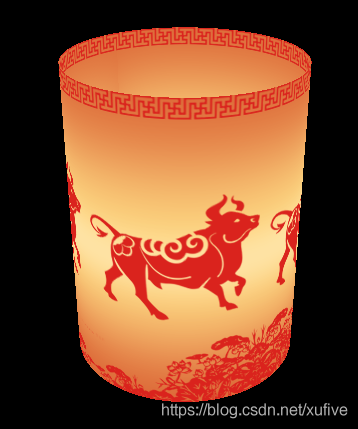``````>>> theta = np.linspace(0, 2*np.pi, 18, endpoint=False)
>>> x = r * np.cos(theta)
>>> y = r * np.sin(theta)
>>> x[2::3] = x[1::3]
>>> x[1::3] = 0
>>> y[2::3] = y[1::3]
>>> y[1::3] = 0
>>> z = np.ones(18) * h * 0.9
>>> vs = np.stack((x,y,z), axis=1)
>>> plt.mesh(xs, ys, zs, im[::-1])
>>> plt.surface(vs, color='#C03000', method='T', mode='FCBC', alpha=0.8)
>>> plt.show()
``````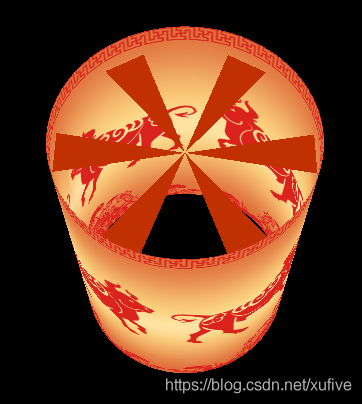``````>>> plt.mesh(xs, ys, zs, im[::-1])
>>> plt.surface(vs, color='#C03000', method='T', mode='FCBC', alpha=0.8)
>>> plt.sphere((0,0,h*0.4), 0.4, '#FFFFFF', slices=60, mode='FCBC')
>>> plt.plot((0,0), (0,0), (0.4*h, 1.5*h), width=3.0, style='solid', cmap='hsv', caxis='z')
``````

``````plt.show(rotation='h-')
``````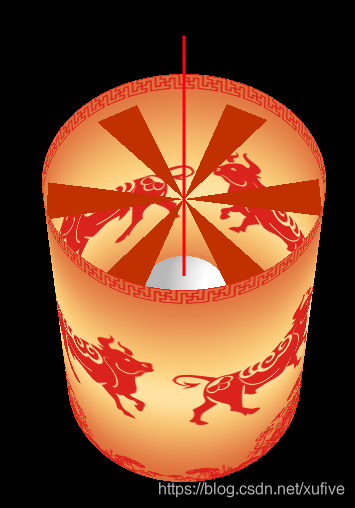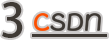``````# -*- coding: utf-8 -*-

import numpy as np
from PIL import Image
import wxgl.wxplot as plt

im = np.array(Image.open('res/paper.png'))/255
rows, cols, deep = im.shape

r, h = 1, 2*np.pi*rows/cols
theta = np.linspace(0, 2*np.pi, cols)
x = r*np.cos(theta)
y = r*np.sin(theta)
z = np.linspace(0, h, rows)
xs = np.tile(x, (rows,1))
ys = np.tile(y, (rows,1))
zs = z.repeat(cols).reshape((rows,cols))

theta = np.linspace(0, 2*np.pi, 18, endpoint=False)
x = r*np.cos(theta)
y = r*np.sin(theta)
x[2::3] = x[1::3]
x[1::3] = 0
y[2::3] = y[1::3]
y[1::3] = 0
z = np.ones(18) * h * 0.9
vs = np.stack((x,y,z), axis=1)

plt.mesh(xs, ys, zs, im[::-1])
plt.surface(vs, color='#C03000', method='T', mode='FCBC', alpha=0.8)
plt.sphere((0,0,h*0.4), 0.4, '#FFFFFF', slices=60, mode='FCBC')
plt.plot((0,0), (0,0), (0.4*h, 1.5*h), width=3.0, style='solid', cmap='hsv', caxis='z')
plt.show(rotation='h-')
``````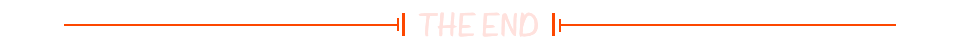``````

☞美团败诉，赔偿饿了么 100 万元；华为 Mate X2 首发秒没；库克获 8 亿年终奖 | 极客头条☞平均月薪 21.8k、中国开发者占比最高，揭晓 Go 语言真实现状！☞拿下丰厚的年终奖，却未能拯救总薪酬，2021 年度 IT 薪酬调查报告出炉！
☞当量子计算遇到机器学习
``````20年前，《新程序员》创刊时，我们的心愿是全面关注程序员成长，中国将拥有新一代世界级的程序员。20年后的今天，我们有了新的使命：助力中国IT技术人成长，成就一亿技术人！